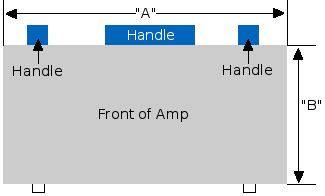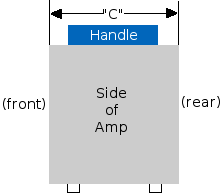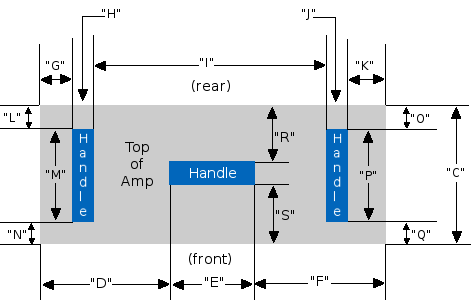Loading... Please wait...

# Combo with 3 Top Handles (AC30)

Send us your measurements
• inches
• inches
• mm
• mm
•• inches
• mm
•• inches
• inches
• inches
*NOTE The dimensions for D + E + F must add up to the measurement for A
• inches
• inches
• inches
• inches
• inches
*NOTE The dimensions for G + H + I + J + K must add up to the measurement for A
• inches
• inches
• inches
*NOTE The dimensions for L + M + N must add up to the measurement for C
• inches
• inches
• inches
*NOTE The dimensions for O + P + Q must add up to the measurement for C
• inches
• inches
• inches
*NOTE The dimensions for R + S + T must add up to the measurement for C
• mm
• mm
• mm
*NOTE The dimensions for D + E + F must add up to the measurement for A
• mm
• mm
• mm
• mm
• mm
*NOTE The dimensions for G + H + I + J + K must add up to the measurement for A
• mm
• mm
• mm
*NOTE The dimensions for L + M + N must add up to the measurement for C
• mm
• mm
• mm
*NOTE The dimensions for O + P + Q must add up to the measurement for C
• mm
• mm
• mm
*NOTE The dimensions for R + S + T must add up to the measurement for C
•//begin script sum text